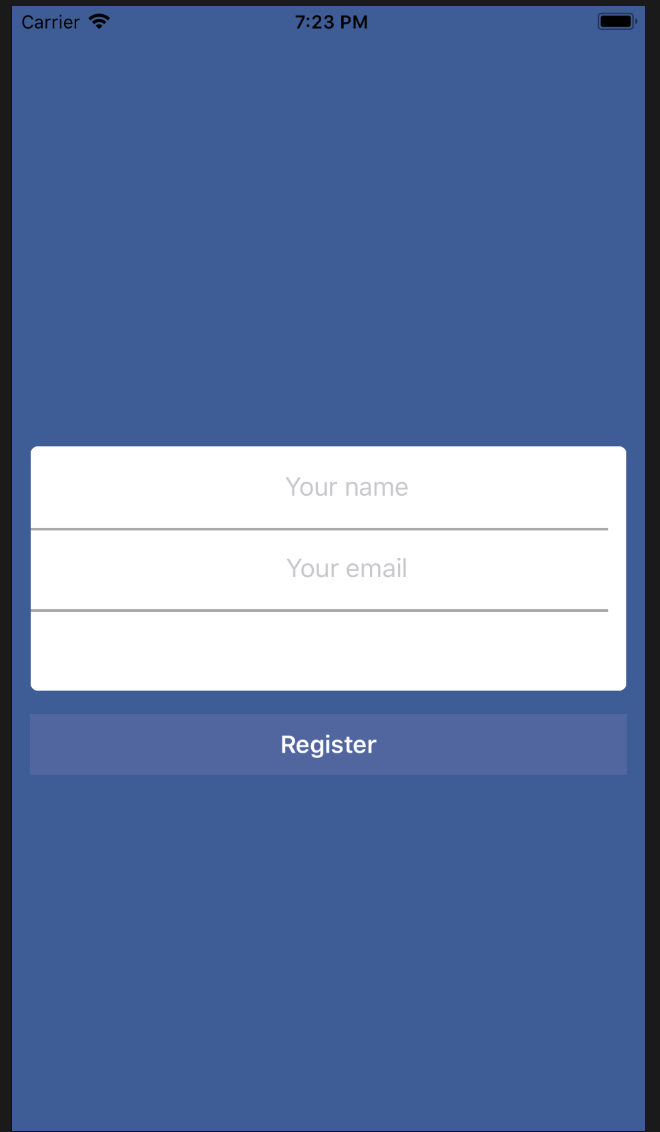#2018 iT 邦幫忙鐵人賽DAY 22
0
Software Development

## DAY: error hen多的一天

UI 介面總算是有個雛形了。``````let nameTextField: UITextField = {
let tf = UITextField()
tf.textAlignment = NSTextAlignment.center

return tf
}()
``````

``````    nameTextField.leftAnchor.constraint(equalTo: inputContainerView.leftAnchor, constant: 12).isActive = true
nameTextField.widthAnchor.constraint(equalTo: inputContainerView.widthAnchor).isActive = true
nameTextField.heightAnchor.constraint(equalTo: inputContainerView.heightAnchor, multiplier: 1 / 3).isActive = true
nameTextField.topAnchor.constraint(equalTo: inputContainerView.topAnchor).isActive = true

``````